# C230 – Solartron RD Appendix A Calculation

## Description

This function calculates the anticipated density and the relative density of the calibration and sample gases.

Solartron 3096 Specific Gravity Transducer Technical Manual – 30960015 July 1989

Solartron 3098 Specific Gravity Transducer Technical Manual – 30985000

R recommended by the Committee on Data for Science and Technology (CODATA) for international use

Kelton calculation reference C230

FLOCALC calculation reference F017

KIMS calculation reference K207

## Options

### Gas Constant R

• Solartron
• AGA 8
• CODATA
• User Entered

Select option based on value you wish to use for the molar gas constant.

### Velocity of Sound Correction

• Simplified
• Entended

Select option based on which velocity of sound correction you wish to employ.

### Transducer Model

• 3096
• 3098

Select option based on the transducer model being used, if applicable.

## Constants

Molecular weight of Methane – Mx = 16.043g/mol

Molecular eight of Nitrogen – My = 28.014 g/mol

Value of gas constant provided by Solartron – 8.31434 Jmol-1K-1

Value of gas constant provided by AGA 8 – 8.31451 Jmol-1K-1

Value of gas constant provided by CODATA – 8.3144621 Jmol-1K-1

## Calculation

All calculations are performed in SI units unless otherwise stated.

### Anticipated density

The anticipated density for each gas is found by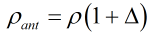Where ρ = Density of gas Δ = Anticipated error

### Velocity of sound correction factor

#### Simplified Version

For Solartron 3096 Specific Gravity Transducer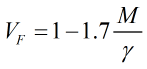Where M = Molecular weight γ = Ratio of specific heats

For Solartron 3098 Specific Gravity Transducer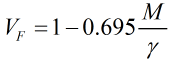Where M = Molecular weight γ = Ratio of specific heats

#### Extended Version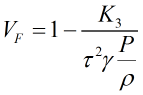Where K3 = Velocity of sound coefficient τ = Sensor periodic time in μs γ = Ratio of specific heats P = Pressure ρ = Density of gas

### Total factor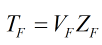Where ZF = Compressibity factor VF = Velocity of sound correction factor

#### Total factor for sample gas using calibration gases as reference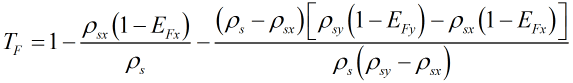Where EFx = Total factor of methane EFy = Total factor of nitrogen ρs = Base density of sample gas ρsx = Base density of methane ρsy = Base density of nitrogen

#### Total factor for nitrogen using methane and sample gas as reference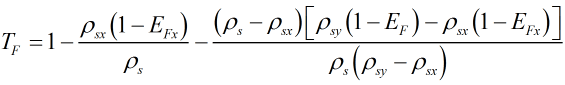Where EFx = Total factor of methane EF = Total factor of sample gas ρs = Base density of sample gas ρsx = Base density of methane ρsy = Base density of nitrogen

Back